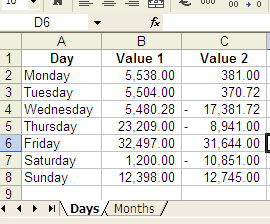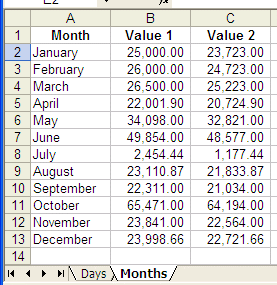# MS Excel and Matlab (interchanging data)

 In this article we'll show how to interchange information between MS Excel and Mathworks' Matlab, working from a Matlab m-file. There are two handy Matlab instructions for this purpose: xlsread and xlswrite.

[num, txt] = xlsread(file, sheet, range)

Where
file = name of xls file (string)
sheet = name of specific sheet within the file (string)
range = specific range to read (string)
num = read numerical data (numeric array or matrix)
txt = read text data (cell array)

Common format for xlswrite (to write an xls.file):

xlswrite(file, m, sheet, range)

Where
m = matrix to insert in xls file
file, sheet and range are as before

An example...

Let's say that we have an Excel file named 'ex2mat.xls' that includes two sheets named 'Days' and 'Months'.

Here's the data for those sheets:We start by setting-up our environment (we assume that the MS Excel .xls file is in the same directory as our Matlab files, otherwise we should take care of the full path for the file under test):

file = 'ex2mat.xls';
sheet1 =
'Days';
sheet2 =
'Months';

[numbers, text] = xlsread(file, sheet1, 'a1:c8')

And we get:

numbers =
1.0e+004 *
0.5538    0.0381
0.5504    0.0371
0.5480   -1.7382
2.3209   -0.8941
3.2497    3.1644
0.1200   -1.0851
1.2398    1.2745

text =
'Day'          'Value 1 '    'Value 2'
'Monday'               ''           ''
'Tuesday'              ''           ''
'Wednesday'            ''           ''
'Thursday'             ''           ''
'Friday'               ''           ''
'Saturday'             ''           ''
'Sunday'               ''           ''

Note that 'text' is a cell array, without numbers.

We can manipulate data and insert the results back in the file (the file must be closed in advance, otherwise Matlab won't update it):

m = mean(numbers)
xlswrite(file, m, sheet1, 'b9:c9')

[numbers, text] = xlsread(file, sheet1, 'a5:c5')
m = m + numbers;
xlswrite(file, m, sheet1,
'b10:c10'

The final result is:We can read the other sheet from the file, like this:

[n1, txt1] = xlsread(file, sheet2, 'a1:c13')
'a2:c2')

And the results are:

n1 =
1.0e+004 *
2.5000    2.3723
2.6000    2.4723
2.6500    2.5223
2.2002    2.0725
3.4098    3.2821
4.9854    4.8577
0.2454    0.1177
2.3111    2.1834
2.2311    2.1034
6.5471    6.4194
2.3841    2.2564
2.3999    2.2722

txt1 =
'Month'        'Value 1 '    'Value 2'
'January'              ''           ''
'February'             ''           ''
'March'                ''           ''
'April'                ''           ''
'May'                  ''           ''
'June'                 ''           ''
'July'                 ''           ''
'August'               ''           ''
'September'            ''           ''
'October'              ''           ''
'November'             ''           ''
'December'             ''           ''

n2 =
25000       23723
txt2 =
'January'

From 'MS Excel' to home

From 'MS Excel' to 'Matlab Programming'

 Top Video: Importing external data